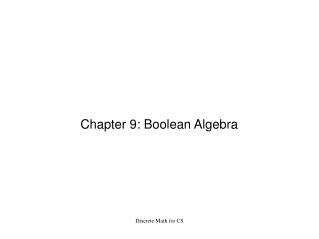DownloadDownload PresentationChapter 9: Boolean Algebra

# Chapter 9: Boolean Algebra

Télécharger la présentation## Chapter 9: Boolean Algebra

- - - - - - - - - - - - - - - - - - - - - - - - - - - E N D - - - - - - - - - - - - - - - - - - - - - - - - - - -
##### Presentation Transcript

1. Chapter 9: Boolean Algebra Discrete Math for CS

2. Discrete Math for CS Disjunction and Conjunction: 0 1 0 1 ∨ ∧ 0 0 1 0 0 0 1 1 1 1 0 1 Our Boolean expressions will use ' to represent negation.

3. Discrete Math for CS Reasoning Laws: • Commutative: p ∧ q = q ∧ p and p ∨ q = q ∨ p • Associative: p ∧ ( q ∧ r ) = ( p ∧ q ) ∧ r p ∨ ( q ∨ r ) = ( p ∨ q ) ∨ r • Distributive: p ∧ ( q ∨ r ) = ( p ∧ q ) ∨(p ∧ r ) p ∨ ( q ∧ r ) = ( p ∨ q ) ∧(p ∨ r )‏ • Idempotent: p ∧ p = p and p ∨ p = p • Absorption: p ∧ ( p ∨ q ) = p and p ∨ ( p ∧ q ) = p • De Morgan: (p ∧ q ) ' = p' ∨ q' and (p ∨ q' ) = p' ∧ q'

4. Discrete Math for CS Exercise: • Prove the Distributive Law p ∧ ( q ∨ r ) = ( p ∧ q ) ∨(p ∧ r )‏

5. Discrete Math for CS Exercise: • Show that (p' ∧ q)' ∧ (p ∨ q) == p (p' ∧ q)' ∧ (p ∨ q) = (p'' ∨ q') ∧ (p ∨ q)‏ = (p ∨ q') ∧ (p ∨ q)‏ = p ∨ (q' ∧ q)‏ = p ∨ 0 = p

6. Discrete Math for CS Boolean Function: • A boolean function, f, on n variables p1, p2, ..., pn is a function f: Bn --> B such that f(p1, p2, ..., pn) is a Boolean expression

7. Discrete Math for CS Minterm: • Suppose a Boolean expression of 3 variables, p, q and r, has only one 1 in its columns of a truth table. This expression is therefore equivalent to a conjunction of p. p', q, q', r and r' that is true for the same row and false elsewhere. p q r f(p,q,r) ... 0 0 1 1 1 ... 0 So f(p,q,r) is equivalent to p' ∧ q ∧ r. Def: A minterm is a Boolean expression that has precisely one 1 in the final column of its truth table. The conjunction taht is equivalent to the minterm is called the product representation of the minterm.

8. Discrete Math for CS Observation: • Any minterm m(p1, p2, ..., pn) can be represented as a conjunction of the variables pi or their negations. • A Boolean expression can be thought of as a disjunction of minterms. Writing it so is putting it in Disjunctive Normal Form.

9. Discrete Math for CS Exercise: • Write the following in Disjunctive Normal Form f(p,q,r) = (p ∧ q) ∨ (q' ∧ r)‏ p q r f 0 0 0 0 0 0 1 1 0 1 0 0 0 1 1 0 1 0 0 0 1 0 1 1 1 1 0 1 1 1 1 1 p' ∧ q' ∧ r p ∧ q' ∧ r p ∧ q ∧ r' p ∧ q ∧ r f(p,q,r) = (p'∧q'∧r) ∨ (p∧q'∧r) ∨ (p∧q∧r') ∨ (p∧q∧r)

10. Discrete Math for CS Complete Set of Operators • A Complete Set of Operators is a set of operators in which you can express every Boolean Expression. • The Disjunctive Normal Form construction shows us that (∨,∧,' ) is a Complete Set of Operators. • Show (∧,' ) is a Complete Set of Operators.

11. Discrete Math for CS • Show NAND is a Complete Set of Operators. • It suffices to show we can write p', p ∨ q and p ∧ q using only NAND. p' = (p ∧ p)' = p NAND p p ∨ q = (p' ∧ q')' = ((p NAND p) ∧ (q NAND q))' = ((p NAND p) NAND (q NAND q))p ∧ q = ((p q)')' = (p NAND q) ' = (p NAND q) NAND (p NAND q)‏